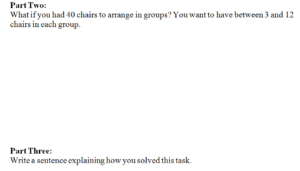Determine the unknown whole number in a multiplication or division equation relating three whole numbers. For example, determine the unknown number that makes the equation true in each of the equations 8 × ? = 48, 5 = [] ÷ 3, 6 × 6 = ?.

Students are able to…

• Write and read related multiplication equations and equations with missing factors.

3×5=15, 15=3×5, 3 x ?=15, 15=3x?, ?x5=15, 15=?x5

• Relate missing factor multiplication equations to division equations using both symbols for division.

Students are able to…because teachers:

• As students become familiar with equation notation connected to their concrete or pictorial representations, give them isolated equations to talk about and describe what the numbers in each group represent. For example, 3×4=12 represents 3 groups of 4 items gives a total of 12 items.
• Include opportunities for students to explore writing equations with missing factors.
• Expect students to build fact families and explain the relationship among the facts, first using multiplication facts with missing factors and the using division facts.

• Give students a basic fact division fact, such as 20 ÷5=4, and ask,  “What are the related multiplication facts?”
• Sample answer that indicates understanding: 5 x 4 =20, 4 x 5 =20
• Give students a basic fact multiplication fact, such as 5×4=20, and ask, “What are the related division facts?”
• Sample answer that indicates understanding: 20 ÷ 5 =4, 20 ÷ 4 =5
• Ask students how the operations of multiplication and division are related.
• Sample answer that indicates understanding:  They are inverse/opposite operations.  In division I know the total and I am trying to solve for the number of groups or things in each group, and in multiplication I know the number of groups and things in each group, but I am trying to find the total.

FSA Notes :

Cognitive Complexity Level: 2 – Basic Application of Skills and Concepts

Achievement Level Descriptors:

2- determines the unknown whole number in a multiplication or division equation, when the unknown number is the product or quotient (with factors and divisors of 1, 2, or 5)

3- determines the unknown whole number in a multiplication or division equation, in any position, with factors and divisors up to 10

4- [intentionally left blank]

5- [intentionally left blank]

Assessment Limits:

All values in items may not exceed whole number multiplication facts of 10 x 10 or the related division facts.

Items must provide the equation.

Students may not be required to create the equation.

Post by Bill McCallum on the difference between OA.1.4 and OA.2.6

Learn Zillion Video: Represent Unknown Numbers Using Symbols or Letters